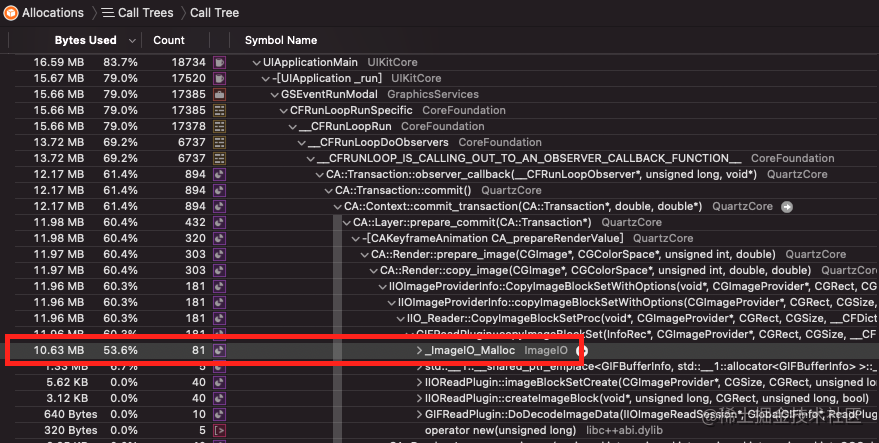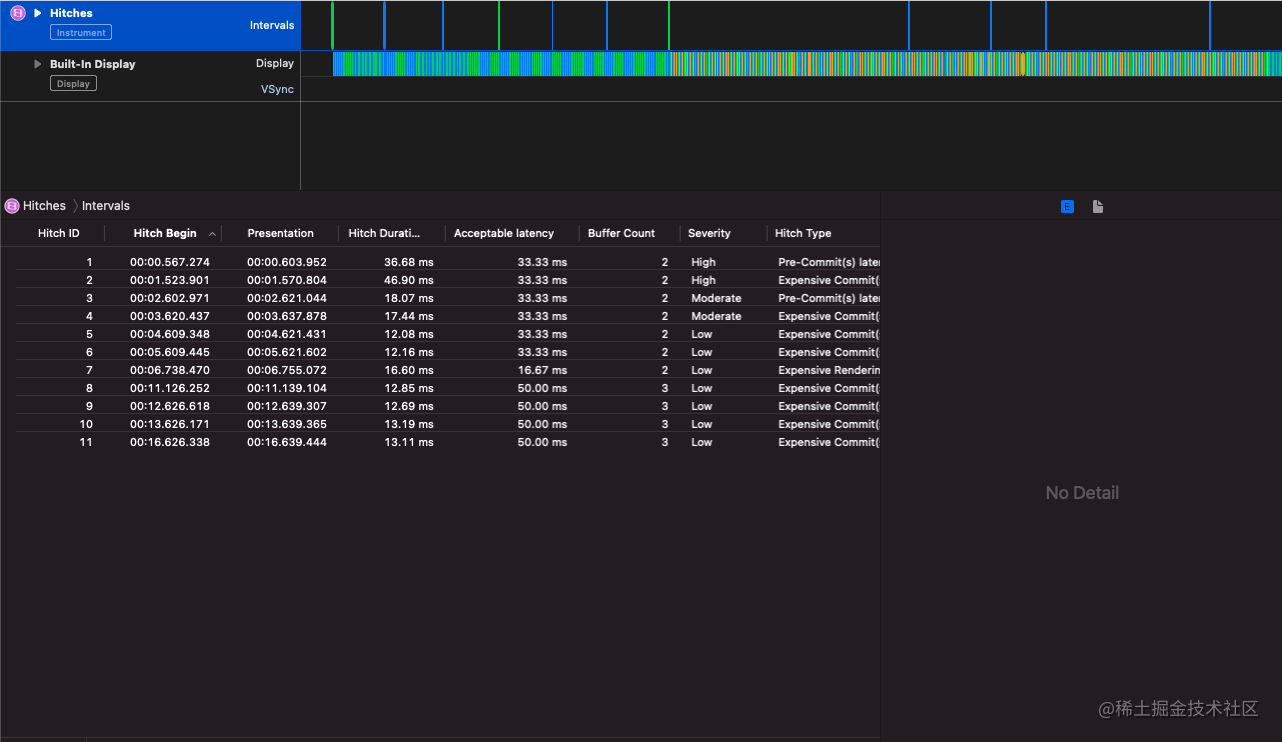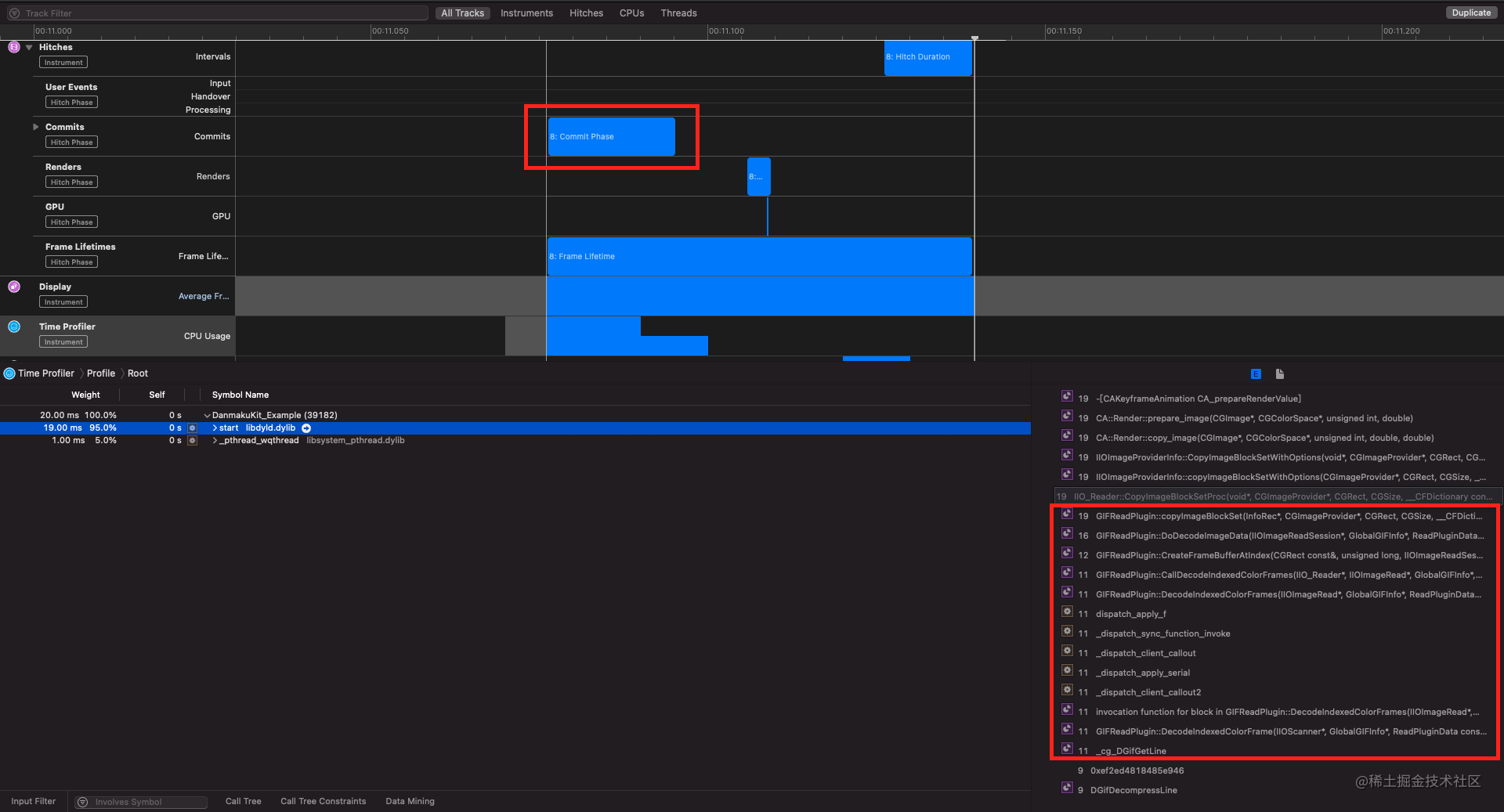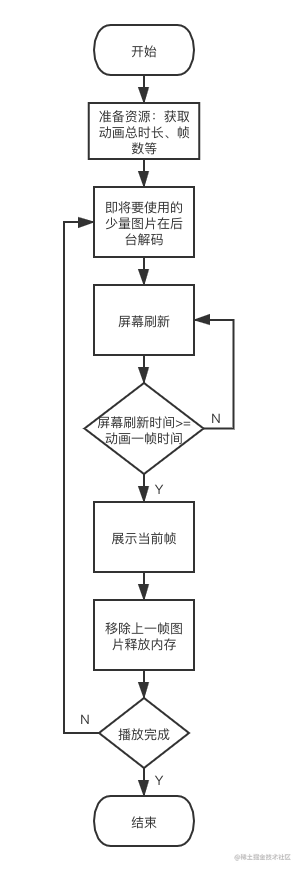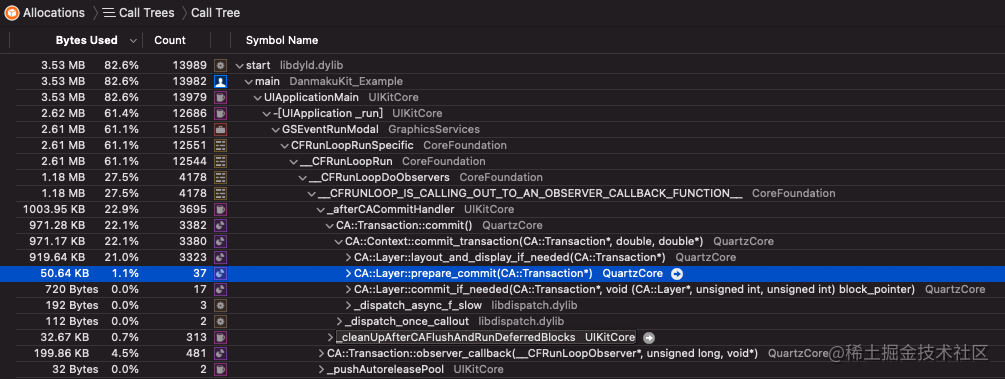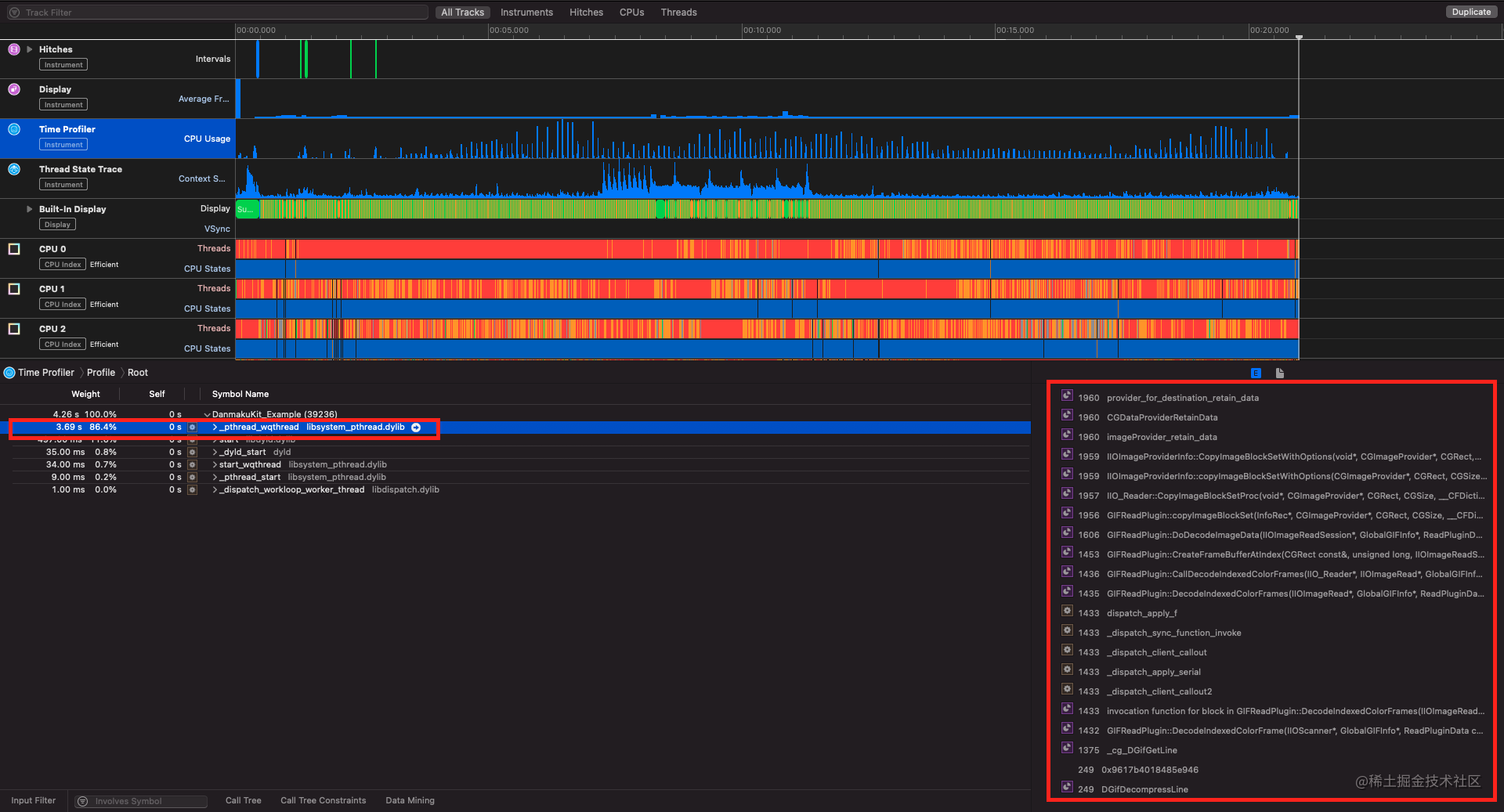# 技术方案

## 帧动画

``````func createGIFAnimation() -> CAKeyframeAnimation? {
guard let data = gifModel?.resource else { return nil }
let bytes = [UInt8](data)
guard let gifData = CFDataCreate(nil, bytes, bytes.count) else { return nil }
guard let image = CGImageSourceCreateWithData(gifData, nil) else { return nil }

var time: Float = 0

var framesArray: [AnyObject] = []
var tempTimesArray: [NSNumber] = []
let frameCount = CGImageSourceGetCount(image)

for i in 0..<frameCount {
var frameDuration: Float = 0.1
let properties = CGImageSourceCopyPropertiesAtIndex(image, i, nil)
guard let framePrpoerties = properties as? [String: AnyObject] else { return nil }
guard let gifProperties = framePrpoerties[kCGImagePropertyGIFDictionary as String] as? [String: AnyObject] else { return nil }

if let delayTimeUnclampedProp = gifProperties[kCGImagePropertyGIFUnclampedDelayTime as String] as? NSNumber {
frameDuration = delayTimeUnclampedProp.floatValue
} else {
if let delayTimeProp = gifProperties[kCGImagePropertyGIFDelayTime as String] as? NSNumber {
frameDuration = delayTimeProp.floatValue
}
}

if frameDuration < 0.011 {
frameDuration = 0.1
}

if let frame = CGImageSourceCreateImageAtIndex(image, i, nil) {
tempTimesArray.append(NSNumber(value: frameDuration))
framesArray.append(frame)
}

time += frameDuration
}

var timesArray: [NSNumber] = []
var base: Float = 0
for duration in tempTimesArray {
base += duration.floatValue / time
timesArray.append(NSNumber(value: base))
}

timesArray.append(NSNumber(value: 1.0))

let animation = CAKeyframeAnimation(keyPath: "contents")
animation.beginTime = CACurrentMediaTime()
animation.duration = CFTimeInterval(time)
animation.repeatCount = Float.greatestFiniteMagnitude
animation.isRemovedOnCompletion = false
animation.fillMode = .forwards
animation.values = framesArray
animation.keyTimes = timesArray
animation.timingFunction = CAMediaTimingFunction(name: .linear)
animation.calculationMode = .discrete
return animation

}